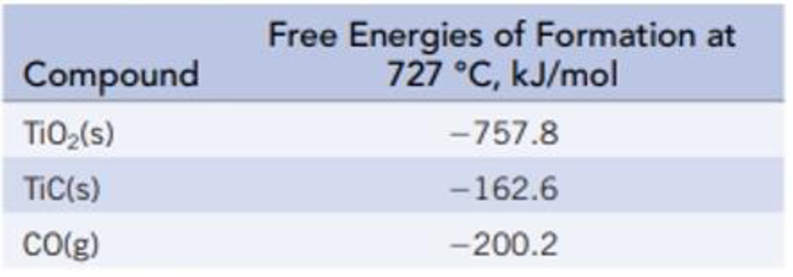# Titanium(IV) oxide is converted to titanium carbide with carbon at a high temperature. TiO 2 (s) + 3 C(s) → 2 CO(g) + TiC(s) (a) Calculate Δ r G ° and K at 727 °C. (b) Is the reaction product-favored at equilibrium at this temperature? (c) How can the reactant or product concentrations be adjusted for the reaction to proceed at 727 °C?### Chemistry & Chemical Reactivity

9th Edition
John C. Kotz + 3 others
Publisher: Cengage Learning
ISBN: 9781133949640

#### Solutions

Chapter
Section### Chemistry & Chemical Reactivity

9th Edition
John C. Kotz + 3 others
Publisher: Cengage Learning
ISBN: 9781133949640
Chapter 18, Problem 65IL
Textbook Problem
49 views

## Titanium(IV) oxide is converted to titanium carbide with carbon at a high temperature.TiO2(s) + 3 C(s) → 2 CO(g) + TiC(s)(a) Calculate ΔrG° and K at 727 °C. (b) Is the reaction product-favored at equilibrium at this temperature? (c) How can the reactant or product concentrations be adjusted for the reaction to proceed at 727 °C?

(a)

Interpretation Introduction

Interpretation:

The ΔrGo value and equilibrium constant for the formation of titanium carbide should be calculated.

Concept introduction:

The Gibbs free energy or the free energy change is a thermodynamic quantity represented by ΔrGo. It can be calculated in a similar manner as entropy and enthalpy.  The expression for the free energy change is:

ΔrG°fG°(products)fG°(reactants)

ΔrGo is related to the equilibrium constant Kp by the equation,

ΔrGo=-RTlnKp

The rearranged expression is,

Kp= e-ΔrGoRT

### Explanation of Solution

The ΔrGo value and the equilibrium constant for the formation of titanium carbide are calculated below.

Given:

The Appendix L referred for the values of standard free energy values.

The given reaction is,

TiO2(s) + 3C(s)2CO(g)+TiC(s)

The ΔrGo for TiO2(s) is 757.8 kJ/mol.

The ΔrGo for C(s) is 0 kJ/mol.

The ΔrGo for CO(g) is 200.2 kJ/mol.

The ΔrGo for TiC(s) is 162.6 kJ/mol.

ΔrG°fG°(products)fG°(reactants)=[[(1 mol TiC(s)/mol-rxn)ΔfG°[TiC(s)]+(2 mol CO(g)/mol-rxn)ΔfG°[CO(g)]]-[(1 mol TiO2(s)/mol-rxn)ΔfG°[TiO2(s)]+(3 mol C(s)/mol-rxn

(b)

Interpretation Introduction

Interpretation:

It should be identified that the reaction will be product favoured at equilibrium at 727oC.

Concept introduction:

The Gibbs free energy or the free energy change is a thermodynamic quantity represented by ΔrGo. It can be calculated in a similar manner as entropy and enthalpy.  The expression for the free energy change is:

ΔrG°fG°(products)fG°(reactants)

ΔrGo is related to the equilibrium constant Kp by the equation,

ΔrGo=-RTlnKp

The rearranged expression is,

Kp= e-ΔrGoRT

(c)

Interpretation Introduction

Interpretation:

The way how the products and reactants for given reaction adjusted in order to proceed at given temperature.

Concept introduction:

The Gibbs free energy or the free energy change is a thermodynamic quantity represented by ΔrGo. It can be calculated in a similar manner as entropy and enthalpy.  The expression for the free energy change is:

ΔrG°fG°(products)fG°(reactants)

ΔrGo is related to the equilibrium constant Kp by the equation,

ΔrGo=-RTlnKp

The rearranged expression is,

Kp= e-ΔrGoRT

### Still sussing out bartleby?

Check out a sample textbook solution.

See a sample solution

#### The Solution to Your Study Problems

Bartleby provides explanations to thousands of textbook problems written by our experts, many with advanced degrees!

Get Started

Find more solutions based on key concepts
BMI is of limited value for athletes. pregnant and lactating women adults older than age 65. ah of the above.

Nutrition: Concepts and Controversies - Standalone book (MindTap Course List)

In which domain and kingdom are humans classified?

Biology: The Dynamic Science (MindTap Course List)

Does the cell cycle refer to mitosis as well as meiosis?

Human Heredity: Principles and Issues (MindTap Course List)

What are the two fuels for the urea cycle?

Organic And Biological Chemistry

The average coefficient of linear expansion of copper is 17 106 (C)1. The Statue of Liberty is 93 in tall on a...

Physics for Scientists and Engineers, Technology Update (No access codes included)

Why should there be a tidal bulge on the side of Earth away from the moon?

Oceanography: An Invitation To Marine Science, Loose-leaf Versin